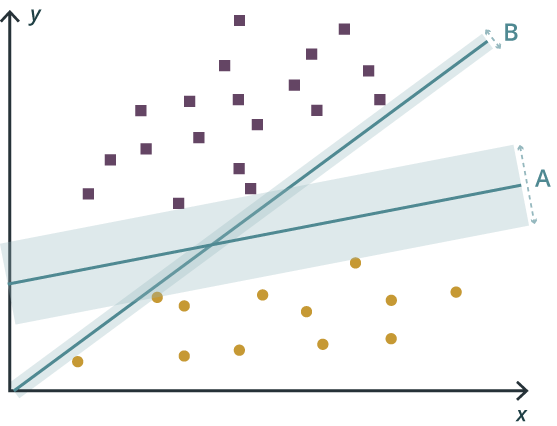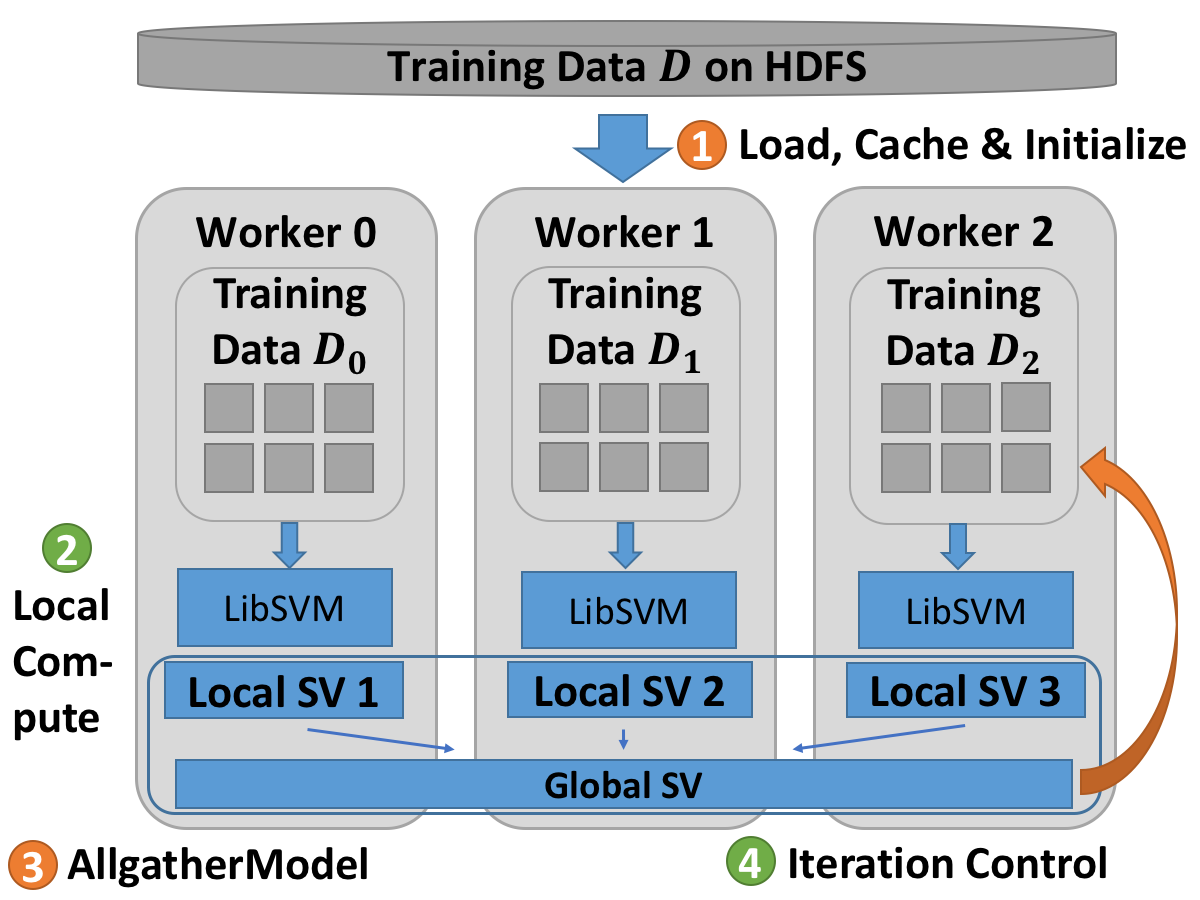Before going through this tutorial take a look at the overview section.In machine learning, support vector machines (SVM) are supervised learning models with associated learning algorithms that analyze data used for classification and regression analysis. Given a set of training examples, each is marked as belonging to one or the other of two categories, an SVM training algorithm builds a model that assigns new examples to one category or the other, making it a non-probabilistic binary linear classifier. An SVM model is a representation of the examples as points in space, mapped so that the examples of the separate categories are divided by a clear gap that is as wide as possible. New examples are then mapped into that same space and are predicted to belong to a category based on which side of the gap they fall.

In addition to performing linear classification, SVMs can efficiently perform a non-linear classification using what is called the kernel trick, implicitly mapping their inputs into high-dimensional feature spaces.

In this tutorial, Harp won’t touch the core code base of computing SVM. It will use LibSVM, which is an open source library and does parallel around LibSVM. And it is developed at the National Taiwan University and written in C++ with other programming languages’ APIs. LibSVM implements the SMO algorithm for kernelized support vector machines (SVMs), supporting classification and regression.

## METHOD

The Harp based SVM algorithm works as follows:

1. The training set of the algorithm is split into subsets.

2. Each node trains sub dataset locally via LibSVM’s API.

3. Allgather support vectors to global.

4. Each node combines its training data and the global support vectors.

5. Repeat Step 2 to 4 until support vectors don’t change any more.The source code can be found in Harp GitHub repository by click Harp SVM.

## Step 0 — Data preprocessing

Harp SVM will follow LibSVM’s data format. Each data point in a file is represented by a line of the format `<label> [<fid>:<feature>]`:

• `<label>` which is 1 or -1
• `<fid>` is a positive feature id
• `<feature>` is the feature value

## Step 1 — Initialize and load data

```Vector<Double> vy = new Vector<Double>();
Vector<svm_node[]> vx = new Vector<svm_node[]>();
Vector<Double> svy = new Vector<Double>();
Vector<svm_node[]> svx = new Vector<svm_node[]>();
int maxIndex = 0;

//read data from HDFS
for (String dataFile : dataFiles) {
FileSystem fs = FileSystem.get(configuration);
Path dataPath = new Path(dataFile);
FSDataInputStream in = fs.open(dataPath);
BufferedReader br = new BufferedReader(new InputStreamReader(in));
String line = "";
while ((line = br.readLine()) != null) {
StringTokenizer st = new StringTokenizer(line," \t\n\r\f:");
vy.addElement(Double.valueOf(st.nextToken()).doubleValue());
int m = st.countTokens() / 2;
svm_node[] x = new svm_node[m];
for (int i = 0;i < m;i++) {
x[i] = new svm_node();
x[i].index = Integer.parseInt(st.nextToken());
x[i].value = Double.valueOf(st.nextToken()).doubleValue();
}
if (m > 0) maxIndex = Math.max(maxIndex,x[m - 1].index);
vx.addElement(x);
}
br.close();
}

HashSet<String> originTrainingData = new HashSet<String>();
for (int i = 0;i < vy.size();i++) {
String line = "";
line += vy.get(i) + " ";
for (int j = 0;j < vx.get(i).length - 1;j++) {
line += vx.get(i)[j].index + ":" + vx.get(i)[j].value + " ";
}
line += vx.get(i)[vx.get(i).length - 1].index + ":" + vx.get(i)[vx.get(i).length - 1].value;
originTrainingData.add(line);
}

//initial svm paramter
svmParameter = new svm_parameter();
svmParameter.svm_type = svm_parameter.C_SVC;
//svmParameter.kernel_type = svm_parameter.RBF;
//svmParameter.degree = 3;
svmParameter.kernel_type = svm_parameter.LINEAR;
svmParameter.gamma = 0;
svmParameter.coef0 = 0;
svmParameter.nu = 0.5;
svmParameter.cache_size = 60000;
svmParameter.C = 1;
svmParameter.eps = 1e-3;
svmParameter.p = 0.1;
svmParameter.shrinking = 1;
svmParameter.probability = 0;
svmParameter.nr_weight = 0;
svmParameter.weight_label = new int;
svmParameter.weight = new double;
```

## Step 2 —Train via LibSVM’s API

```//initial svm problem
svmProblem = new svm_problem();
svmProblem.l = currentTrainingData.size();
svmProblem.x = new svm_node[svmProblem.l][];
svmProblem.y = new double[svmProblem.l];
int id = 0;
for (String line : currentTrainingData) {
StringTokenizer st = new StringTokenizer(line," \t\n\r\f:");
svmProblem.y[id] = Double.valueOf(st.nextToken()).doubleValue();
int m = st.countTokens() / 2;
svm_node[] x = new svm_node[m];
for (int i = 0;i < m;i++) {
x[i] = new svm_node();
x[i].index = Integer.parseInt(st.nextToken());
x[i].value = Double.valueOf(st.nextToken()).doubleValue();
}
svmProblem.x[id] = x;
id++;
}

//compute model
svmModel = svm.svm_train(svmProblem, svmParameter);
```

## Step 3 — Communication among nodes

```Table<HarpString> svTable = new Table(0, new HarpStringPlus());

HarpString harpString = new HarpString();
harpString.s = "";
for (int i = 0;i < svmModel.l;i++) {
harpString.s += svmProblem.y[svmModel.sv_indices[i] - 1] + " ";
for (int j = 0;j < svmModel.SV[i].length - 1;j++) {
harpString.s += svmModel.SV[i][j].index + ":" + svmModel.SV[i][j].value + " ";
}
harpString.s += svmModel.SV[i][svmModel.SV[i].length - 1].index + ":" + svmModel.SV[i][svmModel.SV[i].length - 1].value + "\n";
}
Partition<HarpString> pa = new Partition<HarpString>(0, harpString);
svTable.addPartition(pa);

allreduce("main", "allreduce_" + iter, svTable);

supportVectors = new HashSet<String>();
String[] svString = svTable.getPartition(0).get().get().split("\n");
for (String line : svString) {
if (!supportVectors.contains(line)) {
supportVectors.add(line);
}
}
```

# Run example

### Data

The dataset used is a subset of (MNIST)[https://www.csie.ntu.edu.tw/~cjlin/libsvmtools/datasets/multiclass.html#mnist] with 6000 examples selected.

### Compile

Select the profile related to your hadoop version. For ex: hadoop-2.6.0. Supported hadoop versions are 2.6.0, 2.7.5 and 2.9.0

```cd \$HARP_ROOT_DIR
mvn clean package -Phadoop-2.6.0
```
```cd \$HARP_ROOT_DIR/contrib/target
cp contrib-0.1.0.jar \$HADOOP_HOME
cd \$HADOOP_HOME
cp \$HARP_ROOT_DIR/third_party/libsvm-3.17.jar \$HADOOP_HOME/share/hadoop/mapreduce/
```

### Get the dataset and put data onto hdfs

```wget https://www.csie.ntu.edu.tw/~cjlin/libsvmtools/datasets/multiclass/mnist.t.bz2
bunzip2 mnist.t.bz2
hdfs dfs -mkdir -p /harp-test/svm/data
rm -rf data
mkdir -p data
cd data
split -l 5000 ../mnist.t
cd ..
hdfs dfs -put data /harp-test/svm/
```

### Run

```hadoop jar contrib-0.1.0.jar edu.iu.svm.IterativeSVM <number of mappers> <number of iteration> <work path in HDFS> <local data set path>
```

### Example

```hadoop jar contrib-0.1.0.jar edu.iu.svm.IterativeSVM 2 5 /harp-test/svm nolocalfile
```

Fetch the result:

```hdfs dfs -get /harp-test/svm/out
```

The result is the support vectors.